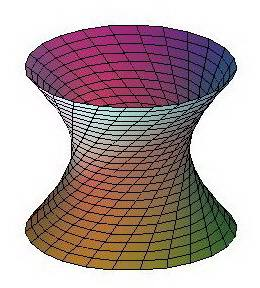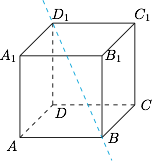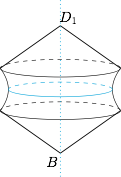每日一题双曲面分析与解　如图，设 $OH$ 是异面直线 $AB,CD$ 的公垂线段，并建立平面直角坐标系 $xOy$．$P$ 点为 $D$ 点在旋转一周的过程中在轴截面 $xOy$ 上的对应点．设 $\overrightarrow{AB}$ 和 $\overrightarrow{CD}$ 的夹角为 $\theta$．由$\overrightarrow{BD}=\overrightarrow{BO}+\overrightarrow{OH}+\overrightarrow{HD},$可得$BD^2=BO^2+OH^2+HD^2-2\cdot BO\cdot HD\cdot\cos\theta,$又 $BO=HD\cdot\cos\theta$，且 $BD=BP$，于是$BP^2-\left(\dfrac{1}{\cos^2\theta}-1\right)BO^2=OH^2,$也即$BP^2-\tan^2\theta\cdot BO^2=OH^2,$此数量关系符合双曲线方程，因此命题得证．如图，考虑到正方体绕直线 $BD_1$ 旋转形成的曲面由圆锥面和双曲面（异面直线绕其中一条旋转形成）构成，因此公共点共有 $5$ 个，为除 $A,B,D_1$ 以外的正方体的顶点．